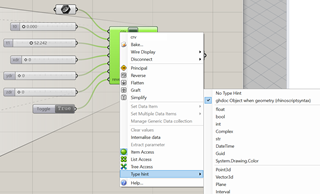# Error: Parameter must be a Guid or string representing a Guid

I was trying to compile a simple component for my grasshopper, to make a subcurve and move the subcurve,the code was successful but when I change the crv input type hint to curve, the error below appears, I can only make the type hint as “ghdoc object when geometry”, but that will lead to the component in grasshopper show the name as “marshalling signal parameter” after compile, anyone know how to resolve the issue?
Thanks!

``````import rhinoscriptsyntax as rs

def msb(curve, domain0, domain1, xaxis, yaxis, zaxis, reverse):
if reverse:
rs.ReverseCurve(curve)
newcurve = rs.FitCurve(curve)
movecurve = rs.MoveObject(subcurve, [float(xaxis), float(yaxis), float(zaxis)])
return movecurve

trimcrv = msb(crv, t0, t1, xdr, ydr, zdr, reverse)

``````

Error: Runtime error (TypeErrorException): Parameter must be a Guid or string representing a Guid

Traceback:
line 890, in coerceguid, “C:\Users\Administrator\AppData\Roaming\McNeel\Rhinoceros\7.0\Plug-ins\IronPython (814d908a-e25c-493d-97e9-ee3861957f49)\settings\lib\rhinoscript\utility.py”
line 3717, in ReverseCurve, “C:\Users\Administrator\AppData\Roaming\McNeel\Rhinoceros\7.0\Plug-ins\IronPython (814d908a-e25c-493d-97e9-ee3861957f49)\settings\lib\rhinoscript\curve.py”
line 6, in msb, “”
line 13, in script

Hi, Caesar

as I understand rhinoscriptsyntax, it does work with the GUID references instead of geometry. rhinoscriptsyntax is a library of python functions. If you want to work with the geometry directly,
use RhinoCommon. See the code below how it would look like if it is written in RhinoCommon:

``````import Rhino.Geometry as rg

# inputs for curve fitting (curve degree and tolerances)
degree = 3
fitTolerance = 0.0001
angleTolerance = 0.0001

if reverse:
curve.Reverse()

# Duplicating curve
subCurve = curve.DuplicateCurve()

# Fitting curve
subCurve = subCurve.Fit(degree, fitTolerance, angleTolerance)

# Createting subcurve from duplicated curve
subCurve = curve.Trim(domain0,domain1)
# Subcurve translation (xaxis, yaxis, zaxis) are vectors
# if floats use
# rg.Transform.Translation(xaxis, yaxis, zaxis)
X_Move = rg.Transform.Translation(xaxis, yaxis, zaxis)
subCurve.Transform(X_Move)
``````

Result in Rhino is a offseted subcurve:

Hope it helps.

Ondřej

1 Like

That is greatly helpful!! Thank you for your solution , I’ll try learn to write in RhinoCommon, it looks easier to be perceived than rhinoscriptsyntax , however, anyone know what is the mistake of my previous code? I am still confused about receiving Error even when I change the input of crv to GUID… must be wrong with the code …

If you set the scriptcontext to the ghdoc, your original code works. See below.

I have noticed this behavior before, but I’m not 100% sure on the cause.

``````import rhinoscriptsyntax as rs
import scriptcontext as sc

sc.doc = ghdoc

def msb(curve, domain0, domain1, xaxis, yaxis, zaxis, reverse):
if reverse:
rs.ReverseCurve(curve)
newcurve = rs.FitCurve(curve)
movecurve = rs.MoveObject(subcurve, [float(xaxis), float(yaxis), float(zaxis)])
return movecurve

crv = rs.coercecurve(crv)
trimcrv = msb(crv, t0, t1, xdr, ydr, zdr, reverse)
``````
2 Likes

Can you send .gh file with the python component which gives you the error?

Ondřej

Here is the file, thank you, I found the situation goes wrong only when reverse is True, maybe something wrong with the line of rs.ReverseCurve(curve)?

MoveSubcurve.gh (9.1 KB)

Hi, I finally come up with a solution with rhino.geometry, everything goes correctly! Thank you!

``````import rhinoscriptsyntax as rs
import Rhino.Geometry as rg

def msb(curve, domain0, domain1, xaxis, yaxis, zaxis, reverse):
if reverse:
rg.Curve.Reverse(curve)
newcurve = rs.FitCurve(curve)
movecurve = rs.MoveObject(subcurve, [float(xaxis), float(yaxis), float(zaxis)])
return movecurve

trimcrv = msb(crv, t0, t1, xdr, ydr, zdr, reverse)

``````

got a solution but still confused about rs.ReverseCurve… don’t know why…but Rhino.Geometry works…

To use rhinoscriptsyntax as in your original script, set the crv input type to ghdoc Object, then return your subcurve.``````import rhinoscriptsyntax as rs

def msb(curve, domain0, domain1, xaxis, yaxis, zaxis, reverse):
if reverse:
rs.ReverseCurve(curve)
newcurve = rs.FitCurve(curve)